# Find 3d distance between 2 sets of points

1965
21
Jump to solution
12-05-2016 02:21 AMby
New Contributor

Hi,

I'd like to find the 3d distance between all points in two point feature classes. One is 12 000 building points, the other 22 000 point locations of a construction machine (along a line), constructing many km of tunnel. My aim is to be able to select the building points within x meters when the construction machine is at a specific location. How can I do this without running Near 3d several thousand times?

I am using arcgis 10.3 with basic license. I am not very familiar with scripting.

Tags (3)
21 RepliesbyEsri Esteemed Contributor

Add a new field (double) and calculate the field using this formula:

``Sqr (([POINT_X_building]-[POINT_X_constructionmachineno2850]^2 +  [POINT_Y_building]-[POINT_Y_constructionmachineno2850]^2 + [POINT_Z_building]-[POINT_Z_constructionmachineno2850]^2 ))‍``byEsri Esteemed Contributor

As an alternative I generated a script that will calculate the 3D distance between two sets of 3D point featureclasses.

The result is a table that contains the OID of the buildings, the OID of the tunnel points and the 3D distance:By defining two relates in ArcMap you will be able to do what you are looking for.

Relate 1: based on the tunnel points, relating to the new table.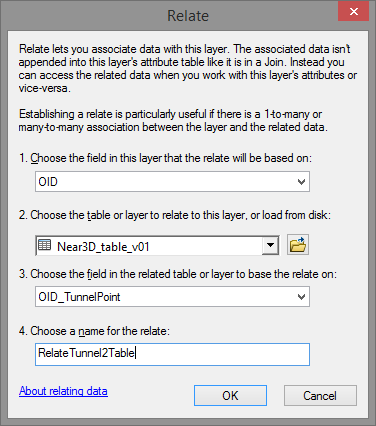Relate 2: based on the table relating to the building points:Make sure to define some attribute indexes in the table to increase the performance: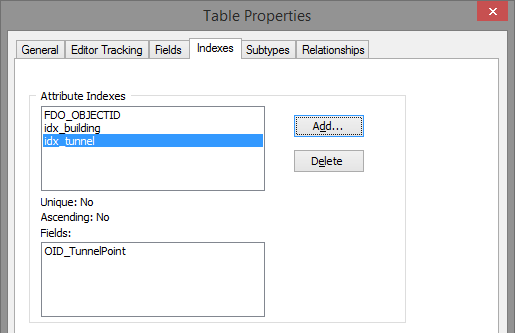How does it work. In ArcMap you select a tunnel point and open the attribute table of the tunnel point featureclass: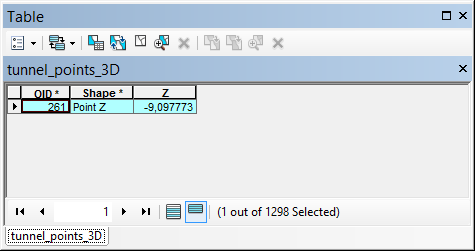Click on the relate button and select the relate: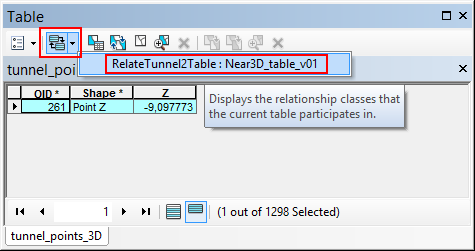This will open the table with the related combinations selected: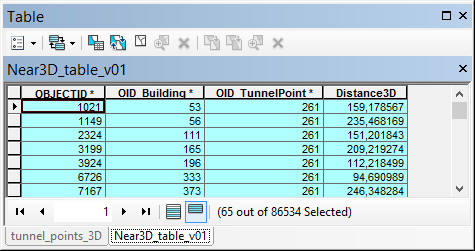Then select the relate to propagate the selection to the building points: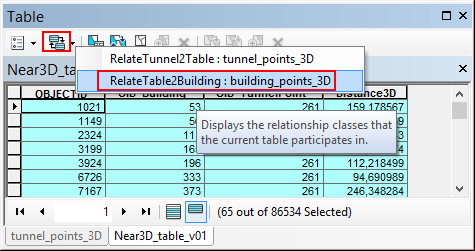... which will open the selected building points:The map will show the selection: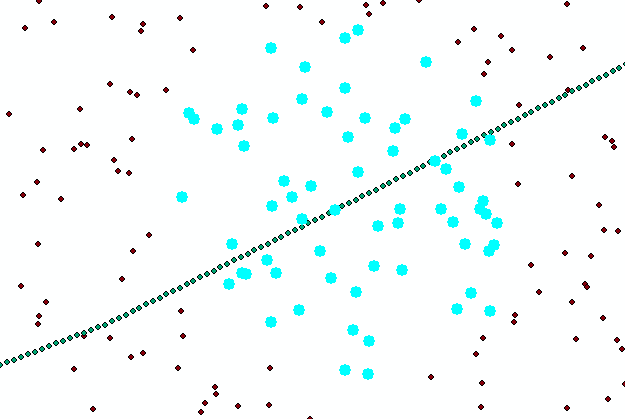The script I used for this is listed below. In my case I only had 6.5M combinations and it took 2 minutes to process the combinations on an average computer. This will probably take all night processing the 264M combinations.

Script:

``import mathimport arcpyfrom time import localtime, strftimedef main():    import os    ShowProgress("Start process...")    fc_buildings = r'D:\Xander\GeoNet\Generate3DNearTable\gdb\data.gdb\building_points_3D'    fc_tunnel_points = r'D:\Xander\GeoNet\Generate3DNearTable\gdb\data.gdb\tunnel_points_3D'    tbl_out = r'D:\Xander\GeoNet\Generate3DNearTable\gdb\data.gdb\Near3D_table_v01'    fld_building_id = 'OID_Building'    fld_tunnel_point_id = 'OID_TunnelPoint'    fld_dist3D = 'Distance3D'    # max distance    max_dist = 250    # sr = arcpy.Describe(fc_buildings).spatialReference    # create lists of buildings and tunnel points    ShowProgress("Create lists of buildings and tunnel points...")    flds = ('OID@', 'SHAPE@')    lst_building = [[r, r] for r in arcpy.da.SearchCursor(fc_buildings, flds)]    lst_tunnelpoints = [[r, r] for r in arcpy.da.SearchCursor(fc_tunnel_points, flds)]    # create output table for relate    ShowProgress("Create output table...")    tbl_ws, tbl_name = os.path.split(tbl_out)    arcpy.CreateTable_management(tbl_ws, tbl_name)    ShowProgress("Add fields to output table...")    AddField(tbl_out, fld_building_id, "LONG", 8)    AddField(tbl_out, fld_tunnel_point_id, "LONG", 8)    AddField(tbl_out, fld_dist3D, "DOUBLE", 8)    cnt_total = len(lst_building) * len(lst_tunnelpoints)    flds = (fld_building_id, fld_tunnel_point_id, fld_dist3D)    ShowProgress("Start creating combinations...")    with arcpy.da.InsertCursor(tbl_out, flds) as curs:        cnt = 0        cnt_found = 0        for building_data in lst_building:            oid_building = building_data            pntg_building = building_data            for tunnel_data in lst_tunnelpoints:                oid_tunnel_point = tunnel_data                pntg_tunnel_point = tunnel_data                cnt += 1                if cnt % 10000 == 0:                    ShowProgressPercentage("Processing combination: {0},  found: {1}".format(cnt, cnt_found), cnt, cnt_total)                dist3D = get3Ddistance(pntg_building, pntg_tunnel_point)                if dist3D <= max_dist:                    cnt_found += 1                    row = (oid_building, oid_tunnel_point, dist3D, )                    curs.insertRow(row)    ShowProgressPercentage("Ready processing {0} combinations,  found: {1}".format(cnt, cnt_found), cnt, cnt_total)def AddField(fc, fld_name, fld_type, fld_length):    if len(arcpy.ListFields(fc, fld_name)) == 0:        arcpy.AddField_management(fc, fld_name, fld_type, None, None, fld_length)def get3Ddistance(pntg1, pntg2):    pnt1 = pntg1.firstPoint    pnt2 = pntg2.firstPoint    distance = math.sqrt((pnt1.X-pnt2.X)**2 + (pnt1.Y-pnt2.Y)**2 + (pnt1.Z-pnt2.Z)**2)    return distancedef ShowProgressPercentage(txt, cnt, cnt_tot):    current_time = strftime("%H:%M:%S", time.localtime())    if cnt_tot is None:        print "{0}   - {1}".format(current_time, txt)    else:        percentage = float(cnt) * 100.0 / float(cnt_tot)        print "{0}   - {1} ({2}%)".format(current_time, txt, round(percentage, 2))def ShowProgress(txt):    # current_time = strftime("%Y-%m-%d %H:%M:%S", gmtime())    current_time = strftime("%H:%M:%S", time.localtime())    print "{0} - {1}".format(current_time, txt)if __name__ == '__main__':    main()‍‍‍‍‍‍‍‍‍‍‍‍‍‍‍‍‍‍‍‍‍‍‍‍‍‍‍‍‍‍‍‍‍‍‍‍‍‍‍‍‍‍‍‍‍‍‍‍‍‍‍‍‍‍‍‍‍‍‍‍‍‍‍‍‍‍‍‍‍‍‍‍‍‍‍‍‍‍‍‍‍‍‍‍‍‍‍‍‍‍‍``by
New Contributor

Thank you so much for spending time on the script, Xander. I ran/started the script with the two point sets and it got to 20% in two hours before I realised that I wanted to split up the point sets, so I just recently started over. I don't think the process will finish before my long weekend starts, but it looks like it's going very well! I appreciate this a lot.by
New Contributor

I have a small problem: Since the objectid in my tunnel point fc is called "objectid" and the new table's objectid is also called "objectid", the tunnel point objectid is not included and cannot be related to the original tunnel point file afterwards. Is there a way of changing the objectid, either choosing another field or renaming it?by
New Contributor

...or can another identifying field be added from the tunnel point attribute table? Each tunnel point has a unique number that is used by the contractors to track the machines, so it needs to be there (either joined or added now)by
New Contributor

(Sorry for spamming)

Nevermind, I just changed what field to include from the tunnel point attribute table, thought it had to be the objectid field. The script works great, I'm very grateful!by
New Contributor

I'm back. Do the fields have to be indexing fields / objectid fields? I used the unique tunnel identifying field now, as mentioned above, and the numbers were changed during the process. So the solution was not as simple as I had hoped. I'm back to wondering if it is possible to change the objectid field name or being able to add a few more fields from the tunnel point attribute table.

Thanks in advance.byEsri Esteemed Contributor

The idea is was to create a table that contains both ObjectID values. Those are read from the input featureclasses. The input ObjectID values won't change and allow you to make the connection between the two featureclasses. The output table contains this relation as would a relationshipclass (which would be available if you would have access to a Standard or Advanced license). It os possible to use a different field as identifier. I used ObjectID since this is standard available in a featureclass. However, this could require to adapt the type of field that is created in the output table.

The alternative could be to join the identifier you want to the output table, using the ObjectID field of the featureclass and the OID_* field in the output table as join fields.

I would suggest to run the process as is and follow the instructions described earlier to see if it works. The advise to create attribute indexes on the fields you use in the relates is to speed up the visualization in ArcMap.byMVP Esteemed Contributor

Xander... do you still have that point file? Not the ridiculously large one, but the option within a selection constraint. I want to do some tests and I don't have a real 3D point file that large with real world coordinates to compare to.  I am testing e_dist (from previous blogs) in 3D.   A post here or email to me would be greatbyEsri Esteemed Contributor

Hi Dan Patterson‌, I have attached a ZIPped GDB that contains the two 3D points files that I used and some intermediate data I used to create the data. The two point featureclasses I used are marked in red: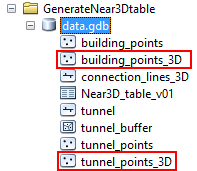I simply drew a line, extracted a point every 10 meters, buffered the line, and generated random points in the buffer polygon, created Z fields with random values and converted the 2D points files to 3D using the attribute.

Very interested to see your results.

Kind regards, Xander